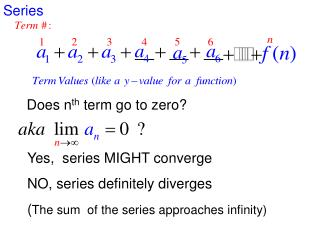DownloadDownload PresentationSeries

# Series

Download Presentation## Series

- - - - - - - - - - - - - - - - - - - - - - - - - - - E N D - - - - - - - - - - - - - - - - - - - - - - - - - - -
##### Presentation Transcript

1. Series Does nth term go to zero? Yes, series MIGHT converge NO, series definitely diverges (The sum of the series approaches infinity)

2. Series Series Series Type: Arithmetic Does nth term go to zero? Therefore, series diverges (The sum of the series approaches infinity)

3. Series Series Type: Arithmetic Does nth term go to zero? Therefore, series diverges (The sum of the series approaches infinity)

4. Series Series Type: Geometric Does nth term go to zero? Therefore, series diverges (The sum of the series approaches infinity)

5. Series Series Type: Geometric Does nth term go to zero? Therefore, the series MIGHT converge (The sum of the series might approach a #)

6. Series Series Type: Geometric Does nth term go to zero? Therefore, the series MIGHT converge (The sum of the series might approach a #)

7. Series Series Type: Harmonic (P-Series with p=1) Does nth term go to zero? Therefore, the series MIGHT converge (The sum of the series might approach a #)

8. Series Series Type: P-Series with p=2 Does nth term go to zero? Therefore, the series MIGHT converge (The sum of the series might approach a #)

9. Series Series Type: P-Series with p=½ Does nth term go to zero? Therefore, the series MIGHT converge (The sum of the series might approach a #)

10. Series Series Type: Telescoping Series Does nth term go to zero? Therefore, the series MIGHT converge (The sum of the series might approach a #)

11. Series Series Type: Telescoping Series Does nth term go to zero? Therefore, the series MIGHT converge (The sum of the series might approach a #)

12. Series Series Type: Telescoping Series Does nth term go to zero? Therefore, the series MIGHT converge (The sum of the series might approach a #)

13. Series Series Type: Telescoping Series Does nth term go to zero? Therefore, the series MIGHT converge (The sum of the series might approach a #)

14. Series Series Type: Alternating Series Does nth term go to zero? Therefore, the series DIVERGES (The sum of the series approaches infinity)

15. Series Series Type: Alternating Harmonic (P-Series p=1) Does nth term go to zero? Therefore, the series MIGHT converge (The sum of the series might approach a #)